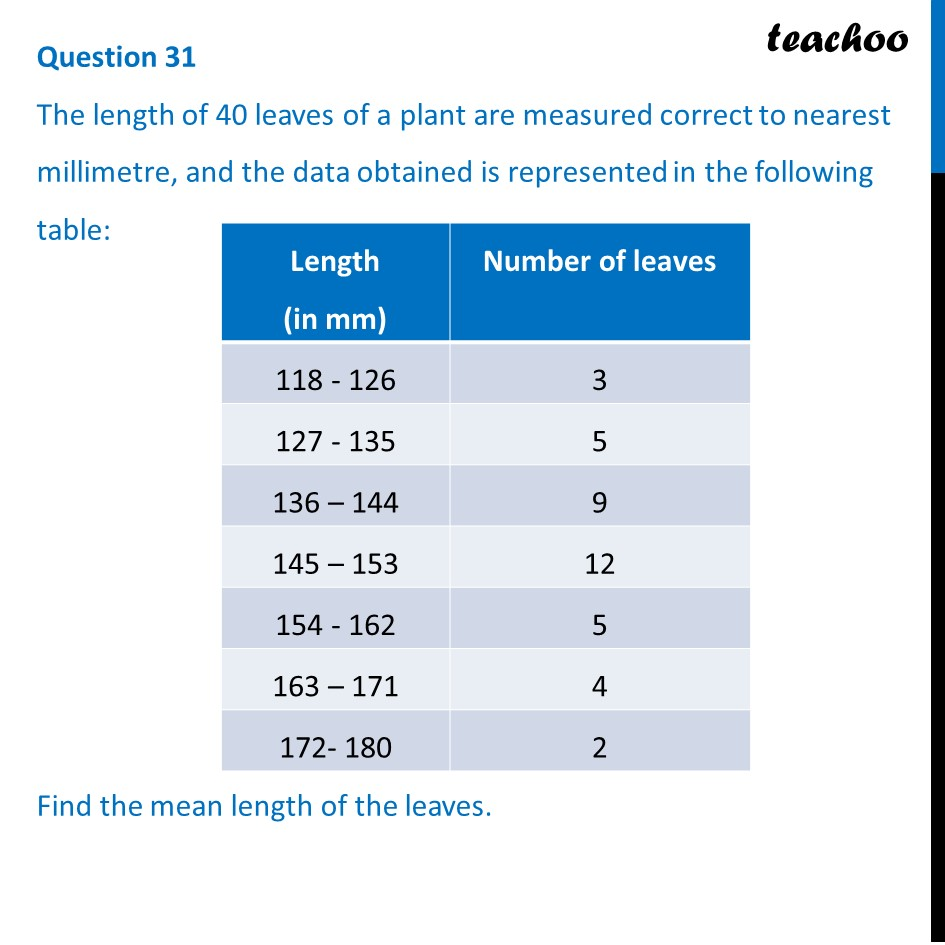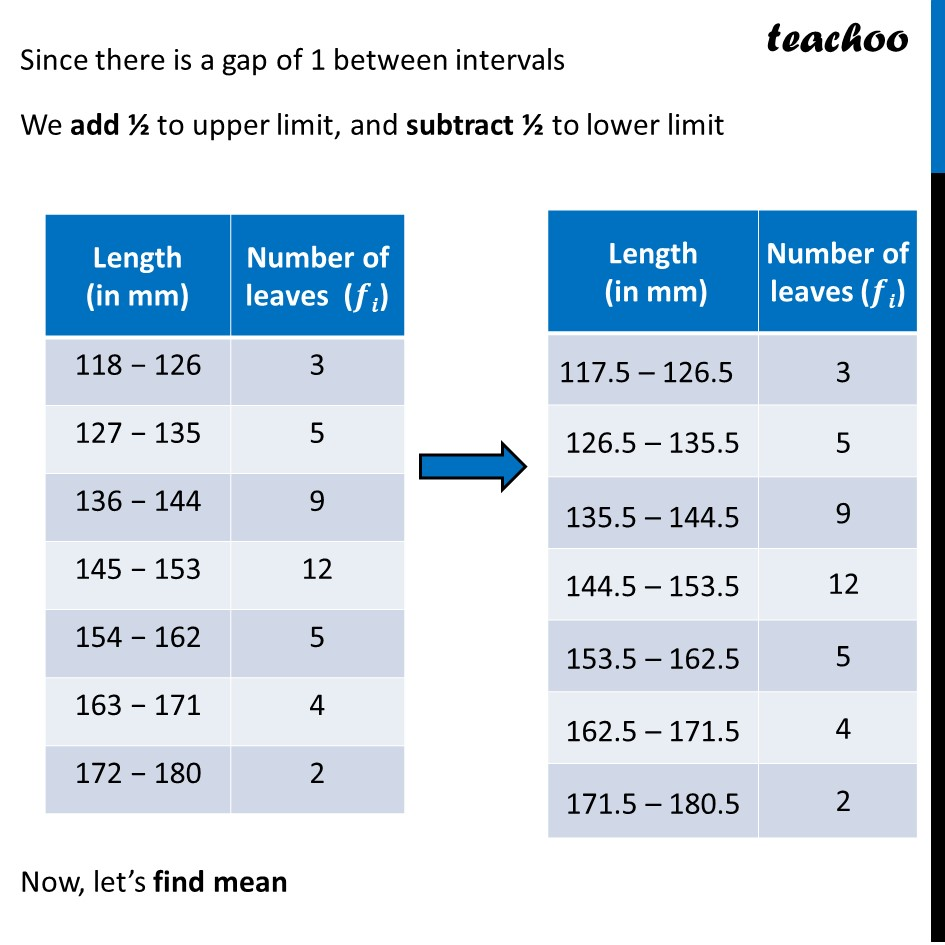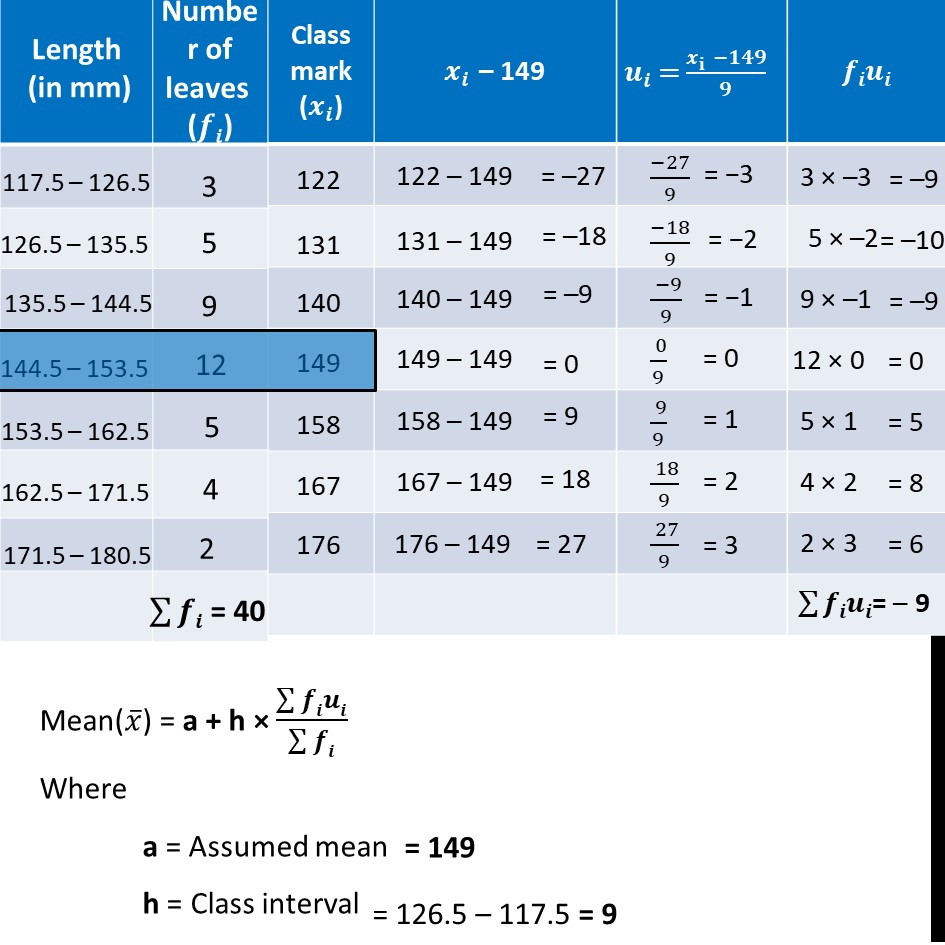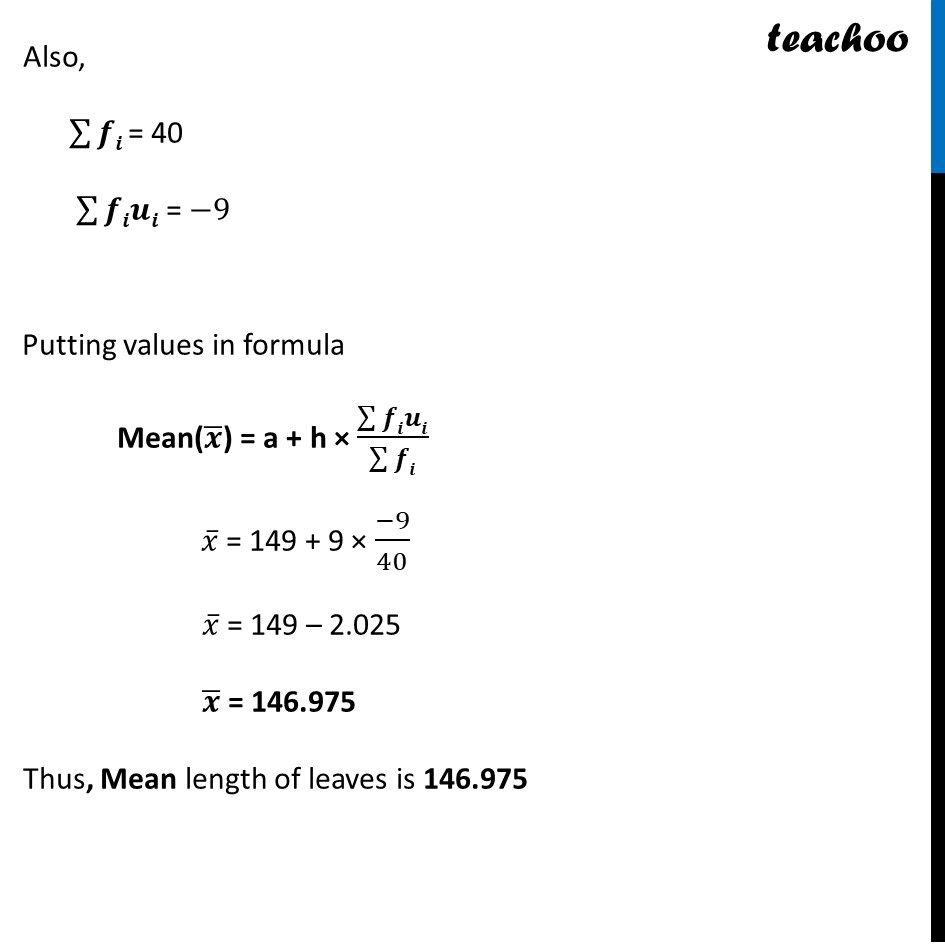CBSE Class 10 Sample Paper for 2024 Boards - Maths Standard

Class 10
Solutions of Sample Papers for Class 10 Boards

## The length of 40 leaves of a plant are measured correct to nearest millimetre, and the data obtained is represented in the following table:

 Length (in mm) Number of leaves 118 - 126 3 127 - 135 5 136 – 144 9 145 – 153 12 154 - 162 5 163 – 171 4 172- 180 2

## Find the mean length of the leaves.

This question is similar to Ex 13.1, 5 Chapter 13 Class 10Learn in your speed, with individual attention - Teachoo Maths 1-on-1 Class

### Transcript

Since there is a gap of 1 between intervals We add ½ to upper limit, and subtract ½ to lower limit Now, let’s find mean Mean(𝑥 ̅) = a + h × (∑▒𝒇𝒊𝒖𝒊)/(∑▒𝒇𝒊) Where a = Assumed mean h = Class interval Also, ∑▒𝒇𝒊 = 40 ∑▒𝒇𝒊𝒖𝒊 = −9 Putting values in formula Mean(𝒙 ̅) = a + h × (∑▒𝒇𝒊𝒖𝒊)/(∑▒𝒇𝒊) 𝑥 ̅ = 149 + 9 × (−9)/40 𝑥 ̅ = 149 – 2.025 𝒙 ̅ = 146.975 Thus, Mean length of leaves is 146.975